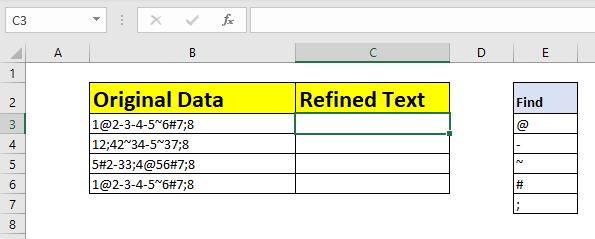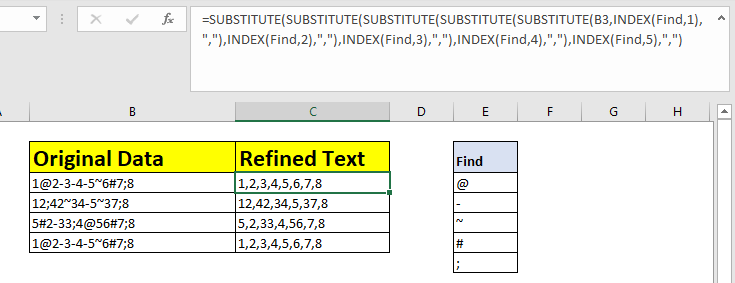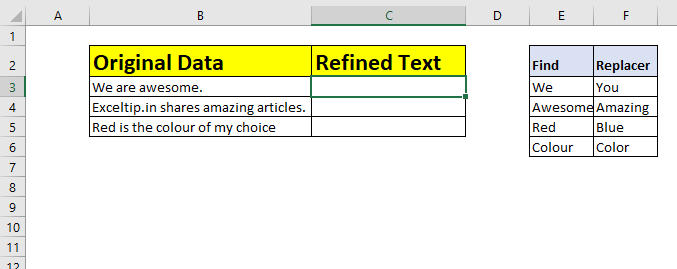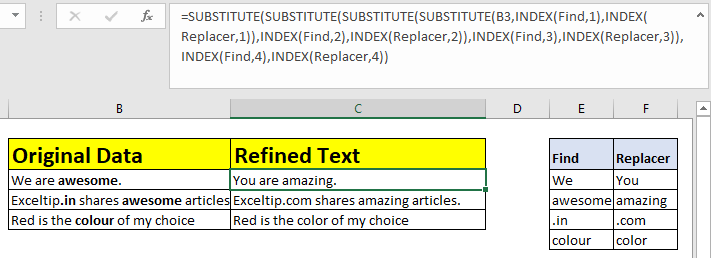# How to Find and Replace Multiple Values in Excel

In this article, we will learn How to Find and Replace Multiple Values in Excel.

Scenario:

We know how to find and replace a single item in the sheet at one time. We just press CTRL+H to open the find and replace dialog and use it to replace a single value. But what if we have multiple items to replace with one or multiple items? How do we replace multiple items in Excel? In this article we will learn exactly that. Hold tight.

Generic Formula in Excel

So we remember the SUBSTITUTE function. We used this function to replace a given text in a string with another text. We will use multiple SUBSTITUTE functions to find multiple values and replace multiple values. The SUBSTITUTE will need help from the INDEX function also. So with that said, let's see the generic formula.

Generic Formula to Find Multiple Items and Replace With One Value

 =SUBSTITUTE(SUBSTITUTE(original_text,INDEX(find_list,1),"replacer_text"),INDEX(find_list,2),"replacer_text")

Generic Formula to Find Multiple Items and Replace With Multiple Values

 =SUBSTITUTE(SUBSTITUTE(original_text,INDEX(find_list,1),INDEX(Replacer_list,1)),INDEX(E3:E7,2),INDEX(Replacer_list,2))

Here

Original_text: This is the reference of the string in which you want to find and replace multiple items.

Find_list: It is a range that contains the list of items to be found. It can be a named range or absolute reference.

Replacer_list (for multiple replace): It is a range that contains the list of items to be replaced with found items. It can be a named range or absolute reference.

1,2… : These are the index of values to find in the find_list and replacer_list.

Replacer_text (for single replacement): When you want to replace multiple items with one single item, in that case you can have a hardcoded text or single cell reference.

The first generic formula is for finding multiple items and replacing it one text at once. The second generic formula is for finding multiple items and replacing them with multiple items at once.

Example :

All of these might be confusing to understand. Let's understand how to use the function using an example. Here we can see in the above image, we have an original data that has some random punctuations between numbers. We have the list of these punctuations in range E3:E7 and have named this range  as Find.

We need to find each character in the Find list and replace it with a comma (,).Since we have to find five characters we will need five nested SUBSTITUTE functions. So write the below formula in B3 and drag down.

 =SUBSTITUTE(SUBSTITUTE(SUBSTITUTE(SUBSTITUTE(SUBSTITUTE(B3,INDEX(find,1),","),INDEX(find,2),","),INDEX(find,3),","),INDEX(find,4),","),INDEX(find,5),",")

Here Find =\$E\$3:\$E\$7You can see that the data is cleaned. We successfully found multiple items of the list and replaced them with a comma. And we have cleaned data with each number separated with a comma.

How does it work?

Well, the formula may look complex but the technique is quite simple. First we replace one text with another and get the replaced string. Now we take this replaced text string as the original string and do the next replacement using another SUBSTITUTE function.

If we talk in the terms of the above example, this is how it is solved.

 =SUBSTITUTE(SUBSTITUTE(SUBSTITUTE(SUBSTITUTE(SUBSTITUTE(B3,"@",","),INDEX(find,2),","),INDEX(find,3),","),INDEX(find,4),","),INDEX(find,5),",") =SUBSTITUTE(SUBSTITUTE(SUBSTITUTE(SUBSTITUTE("1,2-3-4-5~6#7;8","-",","),INDEX(Find,3),","),INDEX(Find,4),","),INDEX(Find,5),",") =SUBSTITUTE(SUBSTITUTE(SUBSTITUTE("1,2,3,4,5~6#7;8","~",","),INDEX(Find,4),","),INDEX(Find,5),",") =SUBSTITUTE(SUBSTITUTE("1,2,3,4,5,6#7;8","#",","),INDEX(Find,5),",") =SUBSTITUTE("1,2,3,4,5,6,7;8",";",",") ="1,2,3,4,5,6,7,8"

Here the INDEX function plays a big role. The INDEX function takes the Find list and in the first iteration (INDEX(find,1)) returns the first value that needs to be found. In the next iteration, it returns the next text that needs to be replaced using INDEX(find,2). And so on. So basically, the INDEX function is used as a feeder to SUBSTITUTE function here that provides values which need to be found and replaced.

## Example 2: Find Multiple Items and Replace with Multiple Values

In the above example we had multiple values to find and replace with one value. In this example, we have multiple values to find and replace them with multiple values.In this example we have a list of items to search and a list of items to replace those items with. How would you do this? You guessed it right. As we used INDEX function to feed the SUBSTITUTE function with find value, we will use INDEX function to feed replacement value too (instead of hardcoded value).

So using the second generic formula we write the below formula in the cell C3 and drag it down.So, how does this work?

It works the same as the first formula. Since we needed to find and replace four values, we have four SUBSTITUTE functions. To find values, we have the same INDEX function. The new thing is another INDEX function, that we use to fetch replacing text.

Let's see how the formula in cell C4 is solved.

 =SUBSTITUTE(SUBSTITUTE(SUBSTITUTE(SUBSTITUTE(B4,"We","You"),INDEX(find,2),INDEX(replacer,2)),INDEX(find,3),INDEX(replacer,3)),INDEX(find,4),INDEX(replacer,4)) =SUBSTITUTE(SUBSTITUTE(SUBSTITUTE("Exceltip.in shares awesome articles.","awesome","amazing"),INDEX(find,3),INDEX(replacer,3)),INDEX(find,4),(replacer,4)) =SUBSTITUTE(SUBSTITUTE("Exceltip.in shares amazing articles.",".in",".com"),INDEX(find,4),INDEX(replacer,4)) =SUBSTITUTE("Exceltip.com shares amazing articles.","colour", "color") =Exceltip.com shares amazing articles.

Here you can see how step by step each substitution is done. The first INDEX pair provides the first find and replacing pair (We and You) and this happens till the last substitution function.

In previous examples, we used the INDEX function only for finding multiple text but in this example we used the INDEX function to fetch replacing text.

The SUBSTITUTE function is case sensitive. If you want a case insensitive formula that finds and replaces multiple values, wrap the original text in LOWER or UPPER function and wrap the old text into the same function.

Formula for finding and replacing multiple items with case insensitive.

 =SUBSTITUTE(SUBSTITUTE(UPPER(B3),UPPER(INDEX(find,1)),","),UPPER(INDEX(find,2)),","))

Here are all the observational notes using the formula in Excel
Notes :

1. The find and replace text should be sorted in a table. As we use indexes to find and replace, make sure that 'find' text has the same index as 'replace' text. It is better to have both lists side by side as we have in the second example.
2. The generic formula has two SUBSTITUTE functions. You will need as many substitute functions as many you have replacements to do.
3. wildcards help with extracting value from the substrings.
4. The COUNTIF function supports logical operators like <, >, <>, = but these are used using double quote sign ( " ) with arguments. But if you are using cell reference for the criteria quotes can be ignored.
5. Criteria argument should use quote sign (") for texts ( like "Gillen" ) or numbers ( like ">50" ).
6. The formula can be used for both texts and numbers.

Hope this article about How to Find and Replace Multiple Values in Excel is explanatory. Find more articles on calculating values and related Excel formulas here. If you liked our blogs, share it with your friends on Facebook. And also you can follow us on Twitter and Facebook. We would love to hear from you, do let us know how we can improve, complement or innovate our work and make it better for you. Write to us at info@exceltip.com.

Related Articles :

Excel REPLACE vs SUBSTITUTE function: The REPLACE and SUBSTITUTE functions are the most misunderstood functions. To find and replace a given text we use the SUBSTITUTE function. Where REPLACE is used to replace a number of characters in string…

Replace text from end of a string starting from variable position: To replace text from the end of the string, we use the REPLACE function. The REPLACE function use the position of text in the string to replace.

How to Check if a string contains one of many texts in Excel: To check if a string contains any of multiple texts, we use this formula. We use the SUM function to sum up all the matches and then perform a logic to check if the string contains any of the multiple strings.

Count Cells that contain specific text: A simple COUNTIF function will do the magic. To count the number of multiple cells that contain a given string we use the wildcard operator with the COUNTIF function.

Popular Articles :